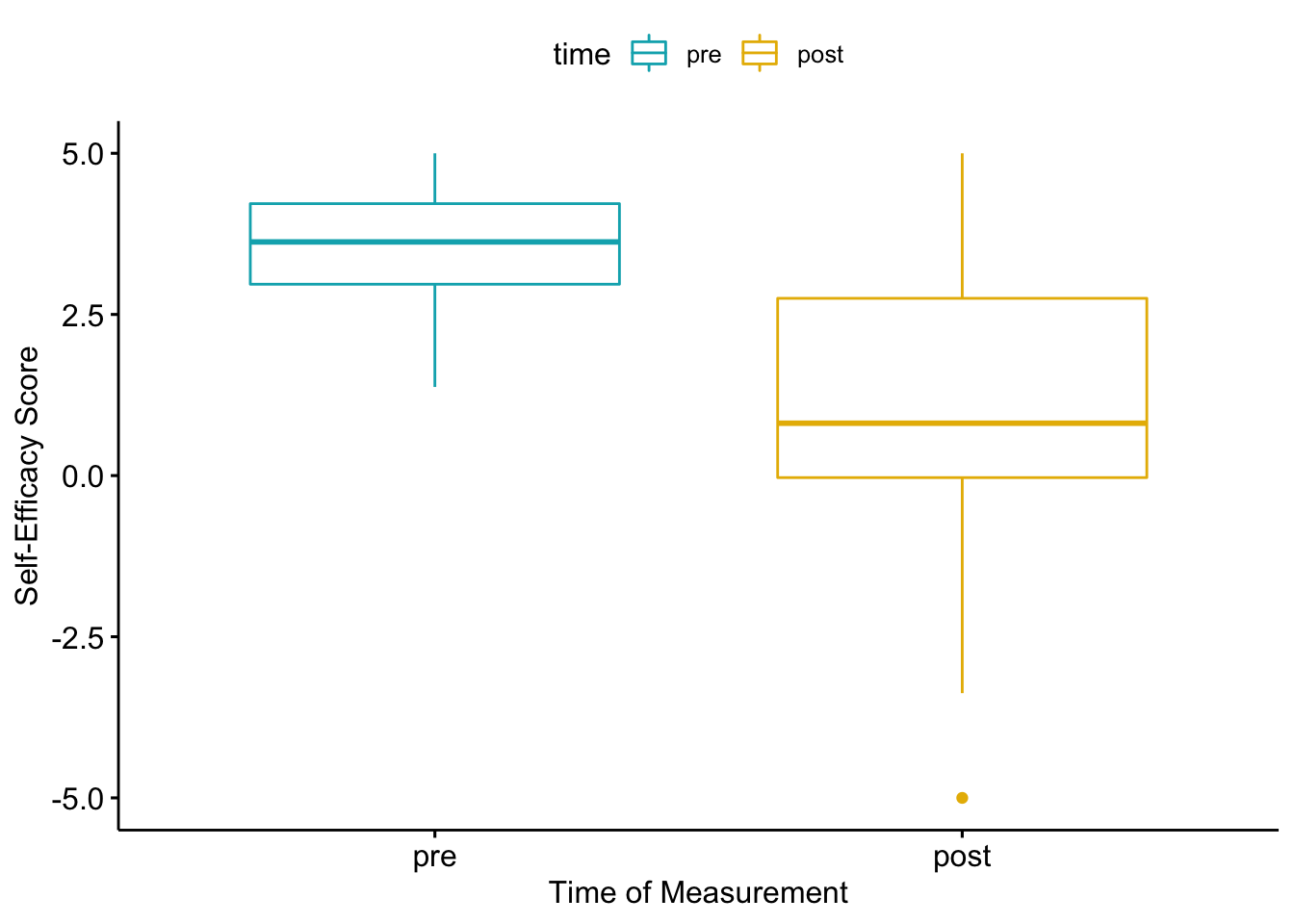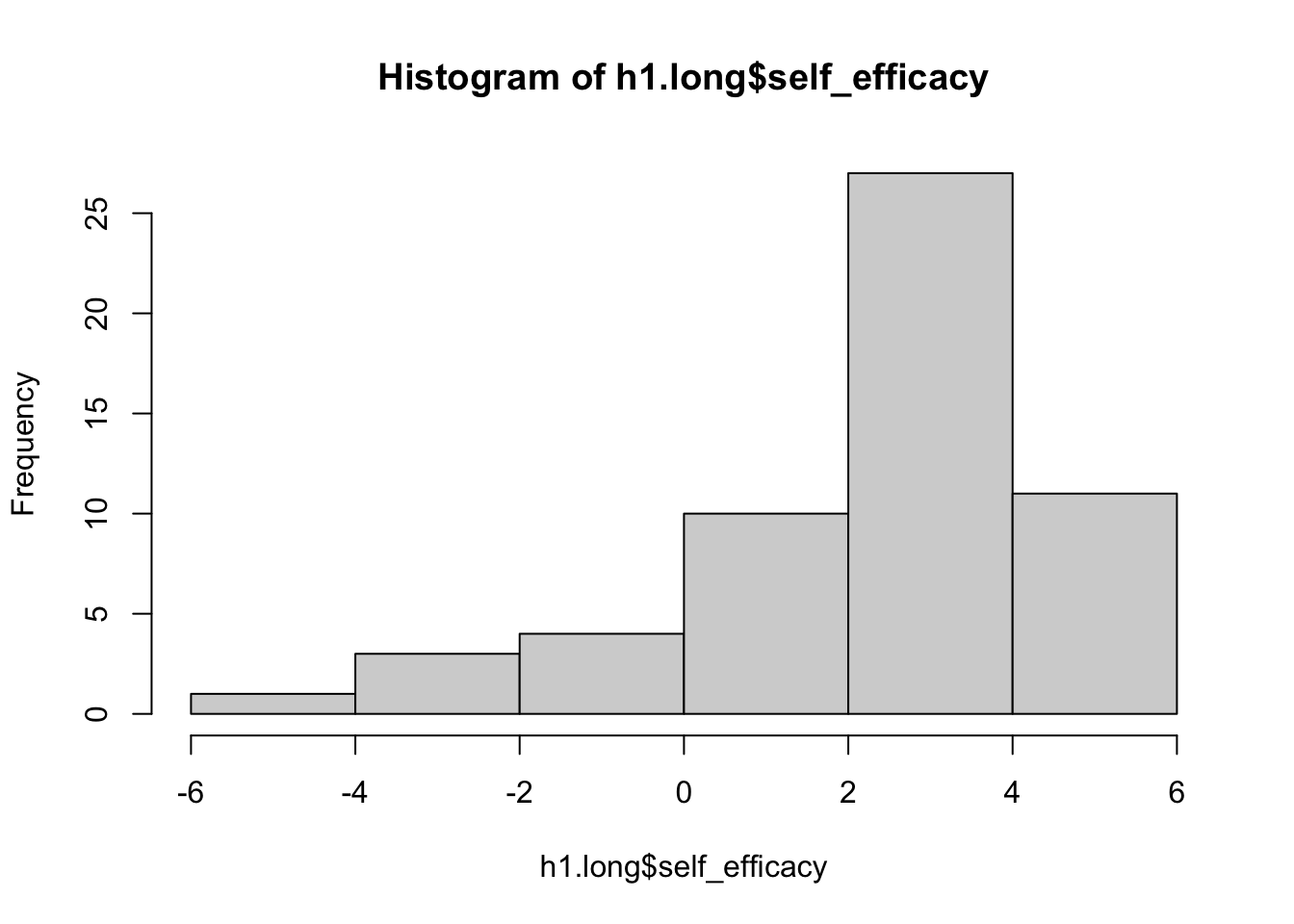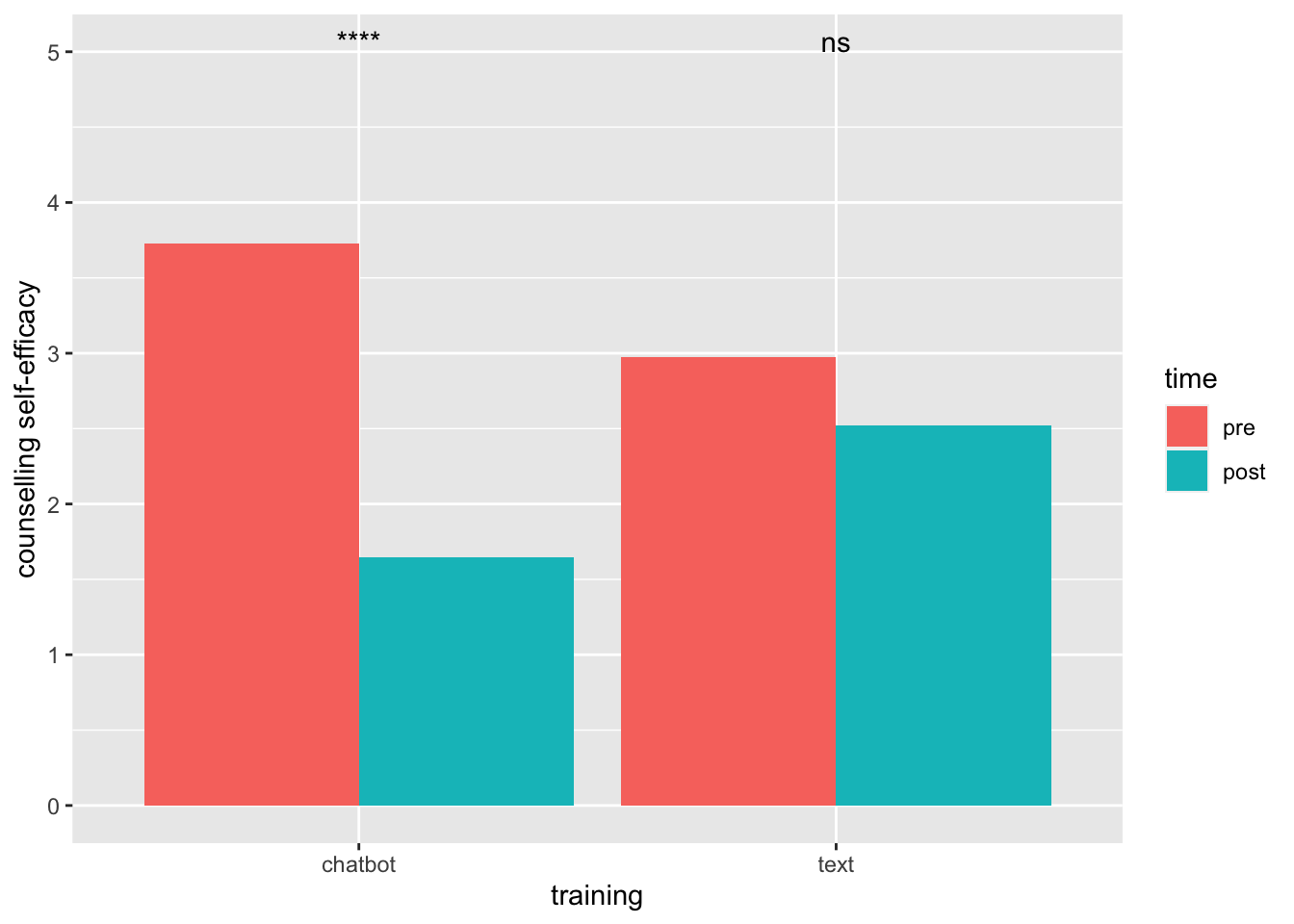## Introduction

This document gives an overview of the analysis of the questionnaires used for evaluating the BDI-based conversational agent developed as part of MSc Thesis “A BDI-based Virtual Agent for Training Child Helpline Counsellors”. The questionnaires include:

• Counselling Self-Efficacy Five Phase Model Questionnaire: measures participants’ self-efficacy towards carrying out tasks related to the Five Phase Model (FPM).

• Perceived Influence on Learning Outcome (PILO): measures participants’ perceived influence of the conversational agent on their knowledge of the Five Phase Model, their attitude towards conversational agents and their self-efficacy through self assessment.

• System Usability Scale (SUS): measures participants’ subjective assessments of usability of the conversational agent.

There are three datasets in total with the following measured variables:

• voormeting: contains the counsellingself-efficacy scores measured pre- and post- the training interventions. This is based on the Counselling Self-Efficacy Five Phase Model Questionnaire.

• nameting: contains the PILO and SUS scores of the participants.

• scores: contains the BDI outcomes of the participants during three training sessions with the coversational agent.

## Libraries

``````library(psych)  # multivariate analysis
library(dplyr)  # data manipulation
library(ggplot2)  # plotting graphs
library(ggpubr)  # plotting graphs
library(tidyverse)  # data manipulation and visualization
library(rstatix)  # pipe-friendly R functions
library(lsmeans)  # linear models
library(multcomp)  # hypothesis testing
# library(plyr)
library(pander)  # markdown``````

``````voormeting = read.csv("/Users/sharongrundmann/Documents/MSc Thesis/Evaluation/Final Experiment/cleaned/voormeting_cleaned.csv",
sep = ";")

sep = ";")

sep = ";")``````

## Hypothesis 1

H1: Training with the conversational agent simulating a child increases the counselling self-efficacy of the participants.

``````# get data
pre <- voormeting[c(14:21)]
pre <- mutate_all(pre, function(x) as.numeric(as.character(x)))

post1 <- voormeting[c(22:29)]
post1 <- mutate_all(post1, function(x) as.numeric(as.character(x)))

post2 <- voormeting[c(33:40)]
post2 <- mutate_all(post2, function(x) as.numeric(as.character(x)))

# find missing values
sum(is.na(pre))``````
``##  0``
``sum(is.na(post1))``
``##  8``
``sum(is.na(post2))``
``##  11``
``````# replace missing row in post1 with zeros
post1[is.na(post1)] = 0

# combine into one dataset
h1 <- data.frame(id = factor(1:28), pre = rowMeans(pre, na.rm = TRUE),
post = rowMeans(post2, na.rm = TRUE))

# put pre and post values in the same column
h1.long <- h1 %>%
gather(key = "time", value = "self_efficacy", pre, post)``````
``````# describe data
h1.long %>%
group_by(time) %>%
get_summary_stats(self_efficacy, show = c("mean", "sd", "median",
"iqr"))``````
``````# boxplot
ggboxplot(h1.long, x = "time", y = "self_efficacy", color = "time",
palette = c("#00AFBB", "#E7B800"), order = c("pre", "post"),
ylab = "Self-Efficacy Score", xlab = "Time of Measurement")````````````# histogram
hist(h1.long\$self_efficacy)````````````# test for normality
d <- with(h1.long, self_efficacy[time == "pre"] - self_efficacy[time ==
"post"])
shapiro.test(d)  # p-value = 0.02679``````
``````##
##  Shapiro-Wilk normality test
##
## data:  d
## W = 0.91543, p-value = 0.02679``````
``````# hence, we cannot assume normality

# wilcox test
res_h1 <- wilcox.test(self_efficacy ~ time, data = h1.long, paired = TRUE)
res_h1``````
``````##
##  Wilcoxon signed rank test with continuity correction
##
## data:  self_efficacy by time
## V = 10, p-value = 2.775e-05
## alternative hypothesis: true location shift is not equal to 0``````
``res_h1\$p.value  # p-value = 2.775e-05``
``##  2.775157e-05``
``qnorm(res_h1\$p.value/2)  # z statistic = -4.191173``
``##  -4.191173``

The p-value of the test is 0.00002775, which is less than the significance level alpha = 0.05. We can then reject null hypothesis and conclude that the average self-efficacy of a participant before training is significantly different from the average self-efficacy after training with a p-value = 0.00002775.

## Hypothesis 2

H2: Training with the conversational agent leads to a higher increase in counselling self-efficacy than the text-based intervention.

``````# get data
a <- subset(voormeting, Group == "A")
b <- subset(voormeting, Group == "B")

a_pre <- a[c(14:21)]
a_post1 <- a[c(22:29)]  # 1 missing row, replace with zeros
a_post1[is.na(a_post1)] = 0
a_post2 <- a[c(33:40)]  # 1 missing value

b_pre <- b[c(14:21)]
b_post1 <- b[c(22:29)]
b_post2 <- b[c(33:40)]  # 10 missing valuess``````

#### H2a

The chatbot training leads to a greater increase in self-efficacy than the text-based training.

``````# h2a
a_chabot_diff = c(rowMeans(a_post1, na.rm = TRUE) - rowMeans(a_pre,
na.rm = TRUE))
a_text_diff = c(rowMeans(a_post2, na.rm = TRUE) - rowMeans(a_post1,
na.rm = TRUE))

b_chatbot_diff = c(rowMeans(b_post2, na.rm = TRUE) - rowMeans(b_post1,
na.rm = TRUE))
b_text_diff = c(rowMeans(b_post1, na.rm = TRUE) - rowMeans(b_pre,
na.rm = TRUE))

# combine dataset
h2a = data.frame(training = rep(c("chatbot", "text"), times = c(11,
17)), difference = c(a_chabot_diff, b_text_diff))

# normality test
shapiro.test(h2a\$difference)``````
``````##
##  Shapiro-Wilk normality test
##
## data:  h2a\$difference
## W = 0.5585, p-value = 4.835e-08``````
``````# describe data
group_by(h2a, training) %>%
dplyr::summarise(count = n(), median = median(difference,
na.rm = TRUE), sd = sd(difference, na.rm = TRUE))``````
``````# wilcox test
res_h2a <- wilcox.test(difference ~ training, data = h2a, alternative = "two.sided")
res_h2a  # p-value = 0.01363``````
``````##
##  Wilcoxon rank sum test with continuity correction
##
## data:  difference by training
## W = 41, p-value = 0.01363
## alternative hypothesis: true location shift is not equal to 0``````

The p-value of the test is 0.01363, which is less than the significance level alpha = 0.05. We conclude that the difference in training is significantly different between the two training interventions.

#### H2b

The chatbot training leads to a greater increase in self-efficacy than the text-based training (increased power).

``````# h2b
h2b = data.frame(id = factor(1:28), chatbot = c(a_chabot_diff,
b_chatbot_diff), text = c(a_text_diff, b_text_diff))

# wilcox test
res_h2b <- wilcox.test(h2b\$chatbot, h2b\$text)
res_h2b  # p-value = 0.0002917``````
``````##
##  Wilcoxon rank sum test with continuity correction
##
## data:  h2b\$chatbot and h2b\$text
## W = 171, p-value = 0.0002917
## alternative hypothesis: true location shift is not equal to 0``````
``````# compute self-efficacy scores based on only present values
chatbot_pre = c(rowMeans(a_pre, na.rm = TRUE), rowMeans(b_post1,
na.rm = TRUE))
chatbot_post = c(rowMeans(a_post1, na.rm = TRUE), rowMeans(b_post2,
na.rm = TRUE))

text_pre = c(rowMeans(a_post1, na.rm = TRUE), rowMeans(b_pre,
na.rm = TRUE))
text_post = c(rowMeans(a_post2, na.rm = TRUE), rowMeans(b_post1,
na.rm = TRUE))

# combine datasets
h2 = data.frame(id = seq.int(28), training = rep(c("chatbot",
"text"), each = 28), pre = c(chatbot_pre, text_pre), post = c(chatbot_post,
text_post))

h2.long <- h2 %>%
gather(key = "time", value = "self_efficacy", pre, post)
h2.long\$time = factor(h2.long\$time, levels = c("pre", "post"))
h2.long\$training = factor(h2.long\$training, levels = c("chatbot",
"text"))``````
``````# anova, simple effect analysis with interventions -
# chatbot, text

# barplot
bar <- ggplot(h2.long, aes(training, self_efficacy, fill = time),
ylab = "counselling self-efficacy") + xlab("training") +
ylab("counselling self-efficacy")
bar + stat_summary(fun = mean, geom = "bar", position = "dodge") +
stat_compare_means(label = "p.signif", method = "wilcox.test",
paired = TRUE)````````````model <- lm(self_efficacy ~ training * time, data = h2.long)
pander(anova(model), caption = "Effect of training, time and interaction effect on counselling self-efficacy")``````
Effect of training, time and interaction effect on counselling self-efficacy
Df Sum Sq Mean Sq F value Pr(>F)
training 1 0.1146 0.1146 0.02996 0.8629
time 1 45.02 45.02 11.77 0.0008518
training:time 1 18.55 18.55 4.852 0.02974
Residuals 108 413 3.824 NA NA
``````h2.long\$simple <- interaction(h2.long\$time, h2.long\$training)  # merge the two factors
levels(h2.long\$simple)``````
``##  "pre.chatbot"  "post.chatbot" "pre.text"     "post.text"``
``````contrastChatbot <- c(1, -1, 0, 0)
contrastText <- c(0, 0, 1, -1)

simpleEff <- cbind(contrastChatbot, contrastText)
contrasts(h2.long\$simple) <- simpleEff

simpleEffectModel <- lm(self_efficacy ~ simple, data = h2.long,
na.action = na.exclude)
pander(summary.lm(simpleEffectModel))``````
Estimate Std. Error t value Pr(>|t|)
(Intercept) 2.719 0.1848 14.71 1.479e-27
simplecontrastChatbot 1.041 0.2613 3.984 0.0001234
simplecontrastText 0.227 0.2613 0.8689 0.3868
simple 0.06397 0.3695 0.1731 0.8629
Fitting linear model: self_efficacy ~ simple Looking at the result, we can see that only a significant difference was found in the contrast Chatbot, and not in contrast Text. These findings seem to confirm our first observation when we look at the figure.
Observations Residual Std. Error \(R^2\) Adjusted \(R^2\)
112 1.955 0.1336 0.1096

We report the results as follows. A linear model was fitted on the counselling self-efficacy of participants, taking the training intervention and time of measurement as independent variables, and including a two-way interaction between these variables. The analysis found a significant main effect (F(1, 27) = 11.77, p < .001) for time but found no significant main effect (F(1, 27), p = 0.86) for training. The analysis also found a significant two-way interaction effect (F(1, 27), p = 0.03) between these two variables. A simple effect analysis further examined the two-way interaction. It revealed a significant (t = 3.98, p < .001) difference in counselling self-efficacy before and after training for the chatbot intervention, but no significant effect (t = 0.87, p = 0.39) was found in the text intervention.

## Hypothesis 3

H3: Participants perceive training with the conversational agent as useful.

``````# pilo analysis

# get data
pilo <- nameting[c(2:9)]
pilo <- mutate_all(pilo, function(x) as.numeric(as.character(x)))  # change to numeric

# find missing values
na_count <- sapply(pilo, function(x) sum(length(which(is.na(x)))))
na_count``````
``````##  Q3_1  Q4_1  Q5_1  Q6_1  Q7_1  Q8_1  Q9_1 Q10_1
##    14    17    15    18    12     5     7     8``````
``````pilo[is.na(pilo)] = 0  # replace missing values with zeros

# normality test
print(apply(pilo, 2, shapiro.test))``````
``````## \$Q3_1
##
##  Shapiro-Wilk normality test
##
## data:  newX[, i]
## W = 0.6051, p-value = 1.183e-07
##
##
## \$Q4_1
##
##  Shapiro-Wilk normality test
##
## data:  newX[, i]
## W = 0.57975, p-value = 5.907e-08
##
##
## \$Q5_1
##
##  Shapiro-Wilk normality test
##
## data:  newX[, i]
## W = 0.73531, p-value = 7.043e-06
##
##
## \$Q6_1
##
##  Shapiro-Wilk normality test
##
## data:  newX[, i]
## W = 0.63748, p-value = 2.997e-07
##
##
## \$Q7_1
##
##  Shapiro-Wilk normality test
##
## data:  newX[, i]
## W = 0.76143, p-value = 1.829e-05
##
##
## \$Q8_1
##
##  Shapiro-Wilk normality test
##
## data:  newX[, i]
## W = 0.94538, p-value = 0.1387
##
##
## \$Q9_1
##
##  Shapiro-Wilk normality test
##
## data:  newX[, i]
## W = 0.94894, p-value = 0.1718
##
##
## \$Q10_1
##
##  Shapiro-Wilk normality test
##
## data:  newX[, i]
## W = 0.93653, p-value = 0.08136``````
``````# we cannot assume normality

# describe data
print(apply(pilo, 2, mean))``````
``````##        Q3_1        Q4_1        Q5_1        Q6_1        Q7_1        Q8_1
## -0.03448276  0.03448276  0.06896552  0.10344828 -0.62068966 -0.48275862
##        Q9_1       Q10_1
## -0.03448276 -1.17241379``````
``print(apply(pilo, 2, sd))``
``````##      Q3_1      Q4_1      Q5_1      Q6_1      Q7_1      Q8_1      Q9_1     Q10_1
## 0.7310833 0.7310833 1.3869554 0.7243138 1.3993313 1.6609096 2.0612541 2.2845594``````